## 4.10Mixed inlet-outlet condition

The inlet-outlet boundary condition is the most basic example of the mixed ﬁxed value/gradient type, described in Sec. 4.9 . The condition sets the reference gradient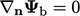and uses a speciﬁed reference value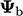. The value fraction is then set to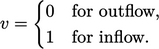(4.11)
The ﬂow direction is established from the sign of the volumetric or mass ﬂux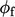at each boundary face, described in Sec. 2.8 , by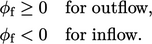(4.12)
The inlet-outlet condition is generally very useful for scalar ﬁelds, e.g. turbulence ﬁelds,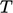, etc. It has an immediate practical use at a free boundary, e.g. in the case introduced in Sec. 4.6 .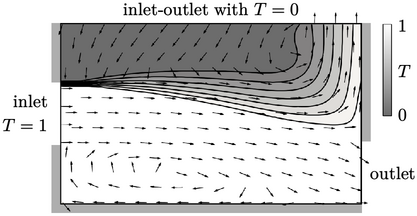The ﬁgure shows the solution of Eq. (2.65 ), converged over time with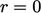and unity Prandtl number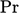, see Sec. 2.21 . The ﬁxed condition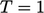is applied at the inlet and a zero gradient condition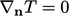at the walls.

At the free boundary, the inlet-outlet condition enables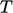to be speciﬁed where inﬂow occurs. The inlet value in the example is set to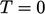; the image shows mixing of ﬂuids at diﬀerent temperatures, from the inlet and entrained at the free boundary.

### Numerical beneﬁt of inlet-outlet

Boundaries may be described “inlet” and “outlet” based on the expectation of the ﬂow direction during a simulation. But the ﬂow direction may not always happen as expected.

In the case of an outlet, for example, inﬂow might occur during a simulation. For example, at the start of a simulation, the initial conditions may induce inﬂow before the internal ﬂow is established. Localised inﬂow can also occur when rotating ﬂow structures pass through an outlet boundary, e.g. when a bluﬀ body sheds vortices, as shown below.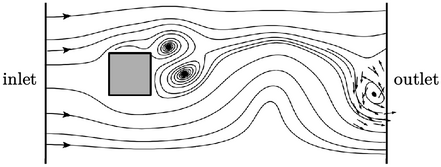Where inﬂow occurs, the inlet-outlet condition can switch to the ﬁxed value type to ensure stability, as discussed in Sec. 4.5 . The inlet-outlet condition is therefore commonly applied to scalar ﬁelds (except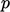), at a boundary which is notionally an outlet, to avoid numerical instability associated with unexpected inﬂow.

Notes on CFD: General Principles - 4.10 Mixed inlet-outlet condition